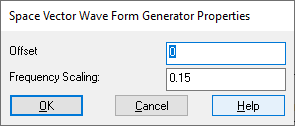## Space Vector Generator (Magnitude/Frequency)

Embedded Category: C2407, F280x

Block Category: TI 16-bit DMC, TI 32-bit DMC

Block Inputs

Frequency: Fractional value to be multiplied by the sample frequency/6 to determine the frequency of the output waveforms (using a frequency scaling of 1).

Gain: Specifies the amplitude of the output waveforms.

Block Output

Va, Vb, Vc: Three-phase voltage outputs suitable for driving PWM-based motor control.

Operating Mode Availability

Simulation mode: YES

C code generation mode: YES

The Space Vector Generator (Magnitude/Frequency) block calculates the appropriate duty ratios needed to generate a given stator reference voltage using space vector PWM technique. The stator reference voltage is described by its α, βcomponents.Frequency Scaling: Indicates sampling rate of the control running on the target.

Offset: Indicates the offset that is used in the calculation of the signal.